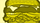## [Filter:Positive Posts] lesley's post body matched "((?<=(\s|\>|\;))|\b)like((?=(\s|\<|\&))|\b)|((?<=(\s|\>|\;))|\b)like\$|^like((?=(\s|\<|\&))|\b)|^like\$", board "Setup".

cancel
Showing results for
Did you mean:Listener

## [Filter:Positive Posts] lesley's post body matched "((?<=(\s|\>|\;))|\b)like((?=(\s|\<|\&))|\b)|((?<=(\s|\>|\;))|\b)like\$|^like((?=(\s|\<|\&))|\b)|^like\$", board "Setup".

[Filter:Positive Posts] lesley's post body matched "((?<=(\s|\>|\;))|\b)like((?=(\s|\<|\&))|\b)|((?<=(\s|\>|\;))|\b)like\$|^like((?=(\s|\<|\&))|\b)|^like\$", board "Setup".

Post Subject: Re: Idea Exchange turned App Exchange - for K2 Developers

Post Body:

I really like this idea, thanks for sharing!

Body text "like" matched filter pattern "((?<=(\s|\>|\;))|\b)like((?=(\s|\<|\&))|\b)|((?<=(\s|\>|\;))|\b)like\$|^like((?=(\s|\<|\&))|\b)|^like\$".

Post by User[id=2721,login=lesley] has message uid 5751.

Community Browser